# CLASS 12 MATHS CHAPTER 1-RELATIONS AND FUNCTIONS

### Solve The Following Questions.

Question1. Determine whether each of the following relations are reflexive, symmetric and transitive:

(i)Relation R in the set A = {1, 2, 3…13, 14} defined as

R = {(x, y): 3x − y = 0}

(ii) Relation R in the set N of natural numbers defined as

R = {(x, y): y = x + 5 and x < 4}

(iii) Relation R in the set A = {1, 2, 3, 4, 5, 6} as

R = {(x, y): y is divisible by x}

(iv) Relation R in the set Z of all integers defined as

R = {(x, y): x − y is as integer}

(v) Relation R in the set A of human beings in a town at a particular time given by

(a) R = {(x, y): x and y work at the same place}

(b) R = {(x, y): x and y live in the same locality}

(c) R = {(x, y): x is exactly 7 cm taller than y}

(d) R = {(x, y): x is wife of y}

(e) R = {(x, y): x is father of y}

Solution :
(i) A = {1, 2, 3 … 13, 14}

R = {(x, y): 3x − y = 0}

∴R = {(1, 3), (2, 6), (3, 9), (4, 12)}

R is not reflexive since (1, 1), (2, 2) … (14, 14) ∉ R.

Also, R is not symmetric as (1, 3) ∈R, but (3, 1) ∉ R. [3(3) − 1 ≠ 0]

Also, R is not transitive as (1, 3), (3, 9) ∈R, but (1, 9) ∉ R.

[3(1) − 9 ≠ 0]

Hence, R is neither reflexive, nor symmetric, nor transitive.

(ii) R = {(x, y): y = x + 5 and x < 4} = {(1, 6), (2, 7), (3, 8)}

It is seen that (1, 1) ∉ R.

∴R is not reflexive. (1, 6) ∈R

But,(6, 1) ∉ R.

∴R is not symmetric.

Now, since there is no pair in R such that (x, y) and (y, z) ∈R, then (x, z) cannot belong to R.

∴ R is not transitive.

Hence, R is neither reflexive, nor symmetric, nor transitive.

(iii) A = {1, 2, 3, 4, 5, 6}

R = {(x, y): y is divisible by x}

We know that any number (x) is divisible by itself.

(x, x) ∈R

∴R is reflexive.

Now, (2, 4) ∈R [as 4 is divisible by 2]

But, (4, 2) ∉ R. [as 2 is not divisible by 4]

∴R is not symmetric.

Let (x, y), (y, z) ∈ R. Then, y is divisible by x and z is divisible by y.

∴z is divisible by x.

⇒ (x, z) ∈R

∴R is transitive.

Hence, R is reflexive and transitive but not symmetric.

(iv) R = {(x, y): x − y is an integer}

Now, for every x ∈ Z, (x, x) ∈R as x − x = 0 is an integer.

∴R is reflexive.

Now, for every x, y ∈ Z if (x, y) ∈ R, then x − y is an integer.

⇒ −(x − y) is also an integer.

⇒ (y − x) is an integer.

∴ (y, x) ∈ R

∴R is symmetric.

Now,

Let (x, y) and (y, z) ∈R, where x, y, z ∈ Z.

⇒ (x − y) and (y − z) are integers.

⇒ x − z = (x − y) + (y − z) is an integer.

∴ (x, z) ∈R

∴R is transitive.

Hence, R is reflexive, symmetric, and transitive.

(v) (a) R = {(x, y): x and y work at the same place}

(x, x) ∈ R

∴ R is reflexive.

If (x, y) ∈ R, then x and y work at the same place.

⇒ y and x work at the same place.

⇒ (y, x) ∈ R.

∴R is symmetric.

Now, let (x, y), (y, z) ∈ R

⇒ x and y work at the same place and y and z work at the same place.

⇒ x and z work at the same place.

⇒ (x, z) ∈R

∴ R is transitive.

Hence, R is reflexive, symmetric, and transitive.

(b) R = {(x, y): x and y live in the same locality}

Clearly (x, x) ∈ R as x and x is the same human being.

∴ R is reflexive.

If (x, y) ∈R, then x and y live in the same locality.

⇒ y and x live in the same locality.

⇒ (y, x) ∈ R

∴R is symmetric.

Now, let (x, y) ∈ R and (y, z) ∈ R.

⇒ x and y live in the same locality and y and z live in the same locality.

⇒ x and z live in the same locality.

⇒ (x, z) ∈ R

∴ R is transitive.

Hence, R is reflexive, symmetric, and transitive.

(c) R = {(x, y): x is exactly 7 cm taller than y}

Now,

(x, x) ∉ R

Since human being x cannot be taller than himself.

∴R is not reflexive.

Now, let (x, y) ∈R.

⇒ x is exactly 7 cm taller than y.

Then, y is not taller than x.

∴ (y, x) ∉R

Indeed if x is exactly 7 cm taller than y, then y is exactly 7 cm shorter than x.

∴R is not symmetric.

Now,

Let (x, y), (y, z) ∈ R.

⇒ x is exactly 7 cm taller thany and y is exactly 7 cm taller than z.

⇒ x is exactly 14 cm taller than z .

∴(x, z) ∉R

∴ R is not transitive.

Hence, R is neither reflexive, nor symmetric, nor transitive.

(d) R = {(x, y): x is the wife of y}

Now,

(x, x) ∉ R

Since x cannot be the wife of herself.

∴R is not reflexive.

Now, let (x, y) ∈ R

⇒ x is the wife of y.

Clearly y is not the wife of x.

∴(y, x) ∉ R

Indeed if x is the wife of y, then y is the husband of x.

∴ R is not symmetric.

Let (x, y), (y, z) ∈ R

⇒ x is the wife of y and y is the wife of z.

This case is not possible. Also, this does not imply that x is the wife of z.

∴(x, z) ∉ R

∴R is not transitive.

Hence, R is neither reflexive, nor symmetric, nor transitive.

(e) R = {(x, y): x is the father of y}

(x, x) ∉ R

As x cannot be the father of himself.

∴R is not reflexive.

Now, let (x, y) ∈R.

⇒ x is the father of y.

⇒ y cannot be the father of y.

Indeed, y is the son or the daughter of y.

∴(y, x) ∉ R

∴ R is not symmetric.

Now, let (x, y) ∈ R and (y, z) ∈ R.

⇒ x is the father of y and y is the father of z.

⇒ x is not the father of z.

Indeed x is the grandfather of z.

∴ (x, z) ∉ R

∴R is not transitive.

Hence, R is neither reflexive, nor symmetric, nor transitive.

Question2. Show that the relation R in the set R of real numbers, defined as

R = {(a, b): a ≤ b2} is neither reflexive nor symmetric nor transitive.

Solution :
R = {(a, b): a ≤ b2}, Relation R is defined as the set of real numbers.

It can be observed that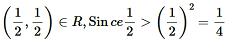∴R is not reflexive.

Now, (1, 4) ∈ R as 1 < 42

But, 4 is not less than 12.

∴(4, 1) ∉ R

∴R is not symmetric.

Now,

(3, 2), (2, 1.5) ∈ R

(as 3 < 22 = 4 and 2 < (1.5)2 = 2.25)

But, 3 > (1.5)2 = 2.25

∴(3, 1.5) ∉ R

∴ R is not transitive.

Hence, R is neither reflexive, nor symmetric, nor transitive.

Question3. Check whether the relation R defined in the set {1, 2, 3, 4, 5, 6} as

R = {(a, b): b = a + 1} is reflexive, symmetric or transitive.

Solution :
Let A = {1, 2, 3, 4, 5, 6}.

A relation R is defined on set A as:

R = {(a, b): b = a + 1}

∴R = {(1, 2), (2, 3), (3, 4), (4, 5), (5, 6)}

We can find (a, a) ∉ R, where a ∈ A.

For instance,

(1, 1), (2, 2), (3, 3), (4, 4), (5, 5), (6, 6) ∉ R

∴R is not reflexive.

It can be observed that (1, 2) ∈ R, but (2, 1) ∉ R.

∴R is not symmetric.

Now, (1, 2), (2, 3) ∈ R

But, (1, 3) ∉ R

∴R is not transitive

Hence, R is neither reflexive, nor symmetric, nor transitive.

Question4. Show that the relation R in R defined as R = {(a, b): a ≤ b}, is reflexive and transitive but not symmetric.

Solution :
R = {(a, b); a ≤ b}

Clearly (a, a) ∈ R as a = a.

∴R is reflexive.

Now,  (2, 4) ∈ R (as 2 < 4)

But, (4, 2) ∉ R as 4 is greater than 2.

∴ R is not symmetric.

Now, let (a, b), (b, c) ∈ R.

Then,  a ≤ b and b ≤ c

⇒ a ≤ c

⇒ (a, c) ∈ R

∴R is transitive.

Hence,R is reflexive and transitive but not symmetric.

Question5. Check whether the relation R in R defined as R = {(a, b): a ≤ b3} is reflexive, symmetric or transitive.

Solution :

Question6. Show that the relation R in the set {1, 2, 3} given by R = {(1, 2), (2, 1)} is symmetric but neither reflexive nor transitive.

Solution :
Let A = {1, 2, 3}.

A relation R on A is defined as R = {(1, 2), (2, 1)}.

It is seen that (1, 1), (2, 2), (3, 3) ∉R.

∴ R is not reflexive.

Now, as (1, 2) ∈ R and (2, 1) ∈ R, then R is symmetric.

Now, (1, 2) and (2, 1) ∈ R

However,  (1, 1) ∉ R

∴ R is not transitive.

Hence, R is symmetric but neither reflexive nor transitive.

Question7. Show that the relation R in the set A of all the books in a library of a college, given by R = {(x, y): x and y have same number of pages} is an equivalence relation.

Solution :
Set A is the set of all books in the library of a college.

R = {x, y): x and y have the same number of pages}

Now, R is reflexive since (x, x) ∈ R as x and x has the same number of pages.

Let (x, y) ∈ R ⇒ x and y have the same number of pages.

⇒ y and x have the same number of pages.

⇒ (y, x) ∈ R

∴R is symmetric.

Now, let (x, y) ∈R and (y, z) ∈ R.

⇒ x and y and have the same number of pages and y and z have the same number of pages.

⇒ x and z have the same number of pages.

⇒ (x, z) ∈ R

∴R is transitive.

Hence, R is an equivalence relation.

Question8. Show that the relation R in the set A = {1, 2, 3, 4, 5} given by R = {(a,b) : |a-b| is even}  is an equivalence relation. Show that all the elements of {1, 3, 5} are related to each other and all the elements of {2, 4} are related to each other. But no element of {1, 3, 5} is related to any element of {2, 4}.

Solution :
A = {1, 2, 3, 4, 5} and R = {(a,b) : |a-b| is even}
, then R = {(1, 3), (1, 5), (3, 5), (2, 4)}

It is clear that for any element a ∈ A , we have|a-a| = 0 (which is even).

∴R is reflexive.

Let (a,b) ∈ R.

=> |a-b| is even

⇒ |-(a-b) | = |b-a| is also even

⇒(b,a) ∈ R

∴R is symmetric.

Now, let (a,b) ) ∈ R and (b, c) ∈ R.

=> |a-b| is even and |b -c| is even

=> (a – b) is even and (b – c) is even

=> (a – c) = (a – b) + (b – c) is even     [Sum of two even integer is even]

=> |a – c | is even

∴R is transitive.

Hence, R is an equivalence relation.

Now, all elements of the set {1, 3, 5} are related to each other as all the elements of this subset are odd. Thus, the modulus of the difference between any two elements will be even.

Similarly, all elements of the set {2, 4} are related to each other as all the elements of this subset are even.

Also, no element of the subset {1, 3, 5} can be related to any element of {2, 4} as all elements of {1, 3, 5} are odd and all elements of {2, 4} are even. Thus, the modulus of the difference between the two elements (from each of these two subsets) will not be even.

Question9. Show that each of the relation R in the set A = {x ∈  Z: 0 ≤  x ≤ 12} ,given by:

(i) R = { (a,b) : |a – b| is a multiple of 4}

(ii) R = { (a,b) : a= b} is an equivalence relation. Find the set of all elements related to 1 in each case.

Solution :

(a) A ={x in Z : 0 <= <= 12} = {0,1,2,3,4,5,6,7,8,9,10,11,12}

R = {(a,b):|a-b| is a multiple of 4}

For any element a  ∈ A, we have (a,a ) ∈ R as |a – a| = 0 is a multiple of 4.

∴R is reflexive.

Now, let (a, b) ∈ R ⇒ |a- b| is a multiple of 4.

=> |-(a-b)|=|b -a| is a multiple of 4

⇒ (b, a ) ∈ R

∴R is symmetric.

Now, let (a,b), (b,c)  ∈ R.

=> |a – b| is multiple of 4 and |b – c| is a multiple of 4

=> (a-b) is a multiple of 4 and (b-c) is a multiple of 4

=> (a-c) = (a – b) + (b – c) is a multiple of 4

=> (a,c)∈ R.

∴ R is transitive.

Hence, R is an equivalence relation.

The set of elements related to 1 is {1, 5, 9} since

|1 – 1| =  0 is a multiple of 4

|5 – 1| =  4 is a multiple of 4

|9 – 1| =  8 is a multiple of 4

(b) R = {(a,b): a = b}

For any element a  ∈ A, we have ( a,a ) ∈ R, since a = a.

∴R is reflexive.

Now, let (a,b ) ∈ R and (b, c) ∈ R.

⇒ a = b and b = c

⇒ a = c

⇒ (a,c) ∈ R.

∴ R is transitive.

Hence, R is an equivalence relation.

The elements in R that are related to 1 will be those elements from set A which are equal to 1.

Hence, the set of elements related to 1 is {1}.

Question10. Given an example of a relation. Which is

(i) Symmetric but neither reflexive nor transitive.

(ii) Transitive but neither reflexive nor symmetric.

(iii) Reflexive and symmetric but not transitive.

(iv) Reflexive and transitive but not symmetric.

(v) Symmetric and transitive but not reflexive.

Solution :
(i) Let A = {5, 6, 7}.

Define a relation R on A as R = {(5, 6), (6, 5)}.

Relation R is not reflexive as (5, 5), (6, 6), (7, 7) ∉ R.

Now, as (5, 6) ∈ R and also (6, 5) ∈ R, R is symmetric.

⇒ (5, 6), (6, 5) ∈ R, but (5, 5) ∉ R

∴R is not transitive.

Hence, relation R is symmetric but not reflexive or transitive.

(ii)Consider a relation R in R defined as:

R = {(a, b): a < b}

For any a ∈ R, we have (a, a) ∉ R since a cannot be strictly less than a itself. In fact, a = a.

∴ R is not reflexive.

Now,  (1, 2) ∈ R (as 1 < 2)

But, 2 is not less than 1.

∴ (2, 1) ∉ R

∴ R is not symmetric.

Now, let (a, b), (b, c) ∈ R.

⇒ a < b and b < c

⇒ a < c

⇒ (a, c) ∈ R

∴R is transitive.

Hence, relation R is transitive but not reflexive and symmetric.

(iii)Let A = {4, 6, 8}.

Define a relation R on A as:

A = {(4, 4), (6, 6), (8, 8), (4, 6), (6, 4), (6, 8), (8, 6)}

Relation R is reflexive since for every a ∈ A, (a, a) ∈R i.e., (4, 4), (6, 6), (8, 8)} ∈ R.

Relation R is symmetric since (a, b) ∈ R ⇒ (b, a) ∈ R for all a, b ∈ R.

Relation R is not transitive since (4, 6), (6, 8) ∈ R, but (4, 8) ∉ R.

Hence, relation R is reflexive and symmetric but not transitive.

(iv) Define a relation R in R as:

R = {a, b): a3 ≥ b3}

Clearly (a, a) ∈ R as a3 = a3.

∴R is reflexive.

Now,

(2, 1) ∈ R (as 23 ≥ 13)

But,

(1, 2) ∉ R (as 13 < 23)

∴ R is not symmetric.

Now,

Let (a, b), (b, c) ∈ R.

⇒ a3 ≥ b3 and b3 ≥ c3

⇒ a≥ c3

⇒ (a, c) ∈ R

∴R is transitive.

Hence, relation R is reflexive and transitive but not symmetric.

(v) Let A = {−5, −6}.

Define a relation R on A as:

R = {(−5, −6), (−6, −5), (−5, −5)}

Relation R is not reflexive as (−6, −6) ∉ R.

Relation R is symmetric as (−5, −6) ∈ R and (−6, −5}∈R.

It is seen that (−5, −6), (−6, −5) ∈ R. Also, (−5, −5) ∈ R.

∴The relation R is transitive.

Hence, relation R is symmetric and transitive but not reflexive.

Question11. Show that the relation R in the set A of points in a plane given by R = {(P, Q): distance of the point P from the origin is same as the distance of the point Q from the origin}, is an equivalence relation. Further, show that the set of all point related to a point P ≠ (0, 0) is the circle passing through P with origin as centre.

Solution :
R = {(P, Q): distance of point P from the origin is the same as the distance of point Q from the origin}

Clearly, (P, P) ∈ R since the distance of point P from the origin is always the same as the distance of the same point P from the origin.

∴R is reflexive.

Now,

Let (P, Q) ∈ R.

⇒ The distance of point P from the origin is the same as the distance of point Q from the origin.

⇒ The distance of point Q from the origin is the same as the distance of point P from the origin.

⇒ (Q, P) ∈ R

∴R is symmetric.

Now,

Let (P, Q), (Q, S) ∈ R.

⇒ The distance of points P and Q from the origin is the same and also, the distance of points Q and S from the origin is the same.

⇒ The distance of points P and S from the origin is the same.

⇒ (P, S) ∈ R

∴R is transitive.

Therefore, R is an equivalence relation.

The set of all points related to P ≠ (0, 0) will be those points whose distance from the origin is the same as the distance of point P from the origin.

In other words, if O (0, 0) is the origin and OP = k, then the set of all points related to P is at a distance of k from the origin.

Hence, this set of points forms a circle with the centre as the origin and this circle passes through point P.

Question12. Show that the relation R defined in the set A of all triangles as R = {(T1, T2): T1 is similar to T2}, is equivalence relation. Consider three right angle triangles T1 with sides 3, 4, 5, T2 with sides 5, 12, 13 and Twith sides 6, 8, 10. Which triangles among T1, T2 and T3 are related?

Solution :
R = {(T1, T2): T1 is similar to T2}

R is reflexive since every triangle is similar to itself.

Further, if (T1, T2) ∈ R, then T1 is similar to T2.

⇒ T2 is similar to T1.

⇒ (T2, T1) ∈R

∴R is symmetric.

Now,

Let (T1, T2), (T2, T3) ∈ R.

⇒ T1 is similar to T2 and T2 is similar to T3.

⇒ T1 is similar to T3.

⇒ (T1, T3) ∈ R

∴ R is transitive.

Thus, R is an equivalence relation.

Now, we can observe that:

Therefore, 3/6 = 4/8 = (5/10 = 1/2)

∴The corresponding sides of triangles T1 and T3 are in the same ratio.

Then, triangle T1 is similar to triangle T3.

Hence, Tis related to T3.

Question13. Show that the relation R defined in the set A of all polygons as R = {(P1, P2): P1 and P2 have same number of sides}, is an equivalence relation. What is the set of all elements in A related to the right angle triangle T with sides 3, 4 and 5?

Solution :
R = {(P1, P2): Pand P2 have same the number of sides}

R is reflexive since (P1, P1) ∈ R as the same polygon has the same number of sides with itself.

Let (P1, P2) ∈ R.

⇒ P1 and P2 have the same number of sides.

⇒ P2 and P1 have the same number of sides.

⇒ (P2, P1) ∈ R

∴R is symmetric.

Now,

Let (P1, P2), (P2, P3) ∈ R.

⇒ P1 and P2 have the same number of sides. Also, P2 and P3 have the same number of sides.

⇒ P1 and P3 have the same number of sides.

⇒ (P1, P3) ∈ R

∴R is transitive.

Hence, R is an equivalence relation.

The elements in A related to the right-angled triangle (T) with sides 3, 4, and 5 are those polygons which have 3 sides (since T is a polygon with 3 sides).

Hence, the set of all elements in A related to triangle T is the set of all triangles.

Question14. Let L be the set of all lines in XY plane and R be the relation in L defined as R = {(L1, L2): L1 is parallel to L2}. Show that R is an equivalence relation. Find the set of all lines related to the line y = 2x + 4.

Solution :
R = {(L1, L2): L1 is parallel to L2}

R is reflexive as any line Lis parallel to itself i.e., (L1, L1) ∈ R.

Now,

Let (L1, L2) ∈ R.

⇒ L1 is parallel to L2.

⇒ L2 is parallel to L1.

⇒ (L2, L1) ∈ R

∴ R is symmetric.

Now,

Let (L1, L2), (L2, L3) ∈R.

⇒ L1 is parallel to L2. Also, L2 is parallel to L3.

⇒ L1 is parallel to L3.

∴R is transitive.

Hence, R is an equivalence relation.

The set of all lines related to the line y = 2x + 4 is the set of all lines that are parallel to the line y = 2x + 4.

Slope of line y = 2x + 4 is m = 2

It is known that parallel lines have the same slopes.

The line parallel to the given line is of the form y = 2x + c, where c ∈R.

Hence, the set of all lines related to the given line is given by y = 2x + c, where c ∈ R.

Question15. Let R be the relation in the set {1, 2, 3, 4} given by R = {(1, 2), (2, 2), (1, 1), (4, 4), (1, 3), (3, 3), (3, 2)}. Choose the correct answer.

(A) R is reflexive and symmetric but not transitive.

(B) R is reflexive and transitive but not symmetric.

(C) R is symmetric and transitive but not reflexive.

(D) R is an equivalence relation.

Solution :
R = {(1, 2), (2, 2), (1, 1), (4, 4), (1, 3), (3, 3), (3, 2)}

It is seen that (a, a) ∈ R, for every a ∈{1, 2, 3, 4}.

∴ R is reflexive.

It is seen that (1, 2) ∈ R, but (2, 1) ∉ R.

∴R is not symmetric.

Also, it is observed that (a, b), (b, c) ∈ R ⇒ (a, c) ∈ R for all a, b, c ∈ {1, 2, 3, 4}.

∴ R is transitive.

Hence, R is reflexive and transitive but not symmetric.

Question16. Let R be the relation in the set N given by R = {(a, b): a = b − 2, b > 6}. Choose the correct answer.

(A) (2, 4) ∈ R (B) (3, 8) ∈R (C) (6, 8) ∈R (D) (8, 7) ∈ R

Solution :
R = {(a, b): a = b − 2, b > 6}

Now, since b > 6, (2, 4) ∉ R

Also, as 3 ≠ 8 − 2, (3, 8) ∉ R

And, as 8 ≠ 7 − 2

(8, 7) ∉ R

Now, consider (6, 8).

We have 8 > 6 and also, 6 = 8 − 2.

∴(6, 8) ∈ R

### Solve The Following Questions.

Question1. Show that the function f : R* → Rdefined by f(x) = 1/x is one-one and onto, where : Ris the set of all non-zero real numbers. Is the result true, if the domain : Ris replaced by N with co-domain being same as : R*?

Solution :

Hence, function g is one-one but not onto.

Question2. Check the injectivity and surjectivity of the following functions:

(i) f: N → N given by f(x) = x2

(ii) f: Z → Z given by f(x) = x

(iii) f: R → R given by f(x) = x2

(iv) f: N → N given by f(x) = x3

(v) f: Z → Z given by f(x) = x3

Solution :
(i) f: N → N is given by,

f(x) = x2

It is seen that for x, y ∈N, f(x) = f(y)

⇒ x2 = y2 ⇒ x = y.

∴f is injective.

Now, 2 ∈ N. But, there does not exist any x in N such that f(x) = x2 = 2.

∴ f is not surjective.

Hence, function f is injective but not surjective.

(ii) f: Z → Z is given by,

f(x) = x2

It is seen that f(−1) = f(1) = 1, but −1 ≠ 1.

∴ f is not injective.

Now,−2 ∈ Z. But, there does not exist any element x ∈Z such that f(x) = x2 = −2.

∴ f is not surjective.

Hence, function f is neither injective nor surjective.

(iii) f: R → R is given by,

f(x) = x2

It is seen that f(−1) = f(1) = 1, but −1 ≠ 1.

∴ f is not injective.

Now,−2 ∈ R. But, there does not exist any element x ∈ R such that f(x) = x2 = −2.

∴ f is not surjective.

Hence, function f is neither injective nor surjective.

(iv) f: N → N given by,

f(x) = x3

It is seen that for x, y ∈N, f(x) = f(y)

⇒ x3 = y3 ⇒ x = y.

∴f is injective.

Now, 2 ∈ N. But, there does not exist any element x in domain N such that f(x) = x= 2.

∴ f is not surjective

Hence, function f is injective but not surjective.

(v) f: Z → Z is given by,

f(x) = x3

It is seen that for x, y ∈ Z, f(x) = f(y)

⇒ x3 = y3 ⇒ x = y.

∴ f is injective.

Now, 2 ∈ Z. But, there does not exist any element x in domain Z such that f(x) = x3 = 2.

∴ f is not surjective.

Hence, function f is injective but not surjective.

Question3. Prove that the Greatest Integer Function f: R → R given by f(x) = [x], is neither one-one nor onto, where [x] denotes the greatest integer less than or equal to x.

Solution :
f: R → R is given by,

f(x) = [x]

It is seen that f(1.2) = [1.2] = 1, f(1.9) = [1.9] = 1.

∴ f(1.2) = f(1.9), but 1.2 ≠ 1.9.

∴ f is not one-one.

Now, consider 0.7 ∈ R.

It is known that f(x) = [x] is always an integer. Thus, there does not exist any element x ∈ R such that f(x) = 0.7.

∴ f is not onto.

Hence, the greatest integer function is neither one-one nor onto.

Question4. Show that the Modulus Function f : R → R, given by f(x) = |x| is neither one-one nor onto, where is |x| if  x is positive or 0 and |-x| is -x  if  x is negative.

Solution :

Question5. Show that the Signum Function f: R → R , given by

is neither one-one nor onto.

Solution :

f: R → R

It is seen that f(1) = f(2) = 1, but 1 ≠ 2.

∴ f  is not one-one.

Now, as f(x) ) takes only 3 values (1, 0, or −1) for the element −2 in co-domain R , there does not exist any x  in domain R  such that

f(x) = −2.

∴ f  is not onto.

Hence, the signum function is neither one-one nor onto.

Question6. Let A = {1, 2, 3}, B = {4, 5, 6, 7} and let f = {(1, 4), (2, 5), (3, 6)} be a function from A to B. Show that f is one-one.

Solution :
It is given that A = {1, 2, 3}, B = {4, 5, 6, 7}.

f: A → B is defined as f = {(1, 4), (2, 5), (3, 6)}.

∴ f (1) = 4, f (2) = 5, f (3) = 6

It is seen that the images of distinct elements of A under f are distinct.

Hence, function f is one-one.

Question7. In each of the following cases, state whether the function is one-one, onto or bijective. Justify your answer.

(i) f: R → R defined by f(x) = 3 − 4x

(ii) f: R → R defined by f(x) = 1 + x2

Solution :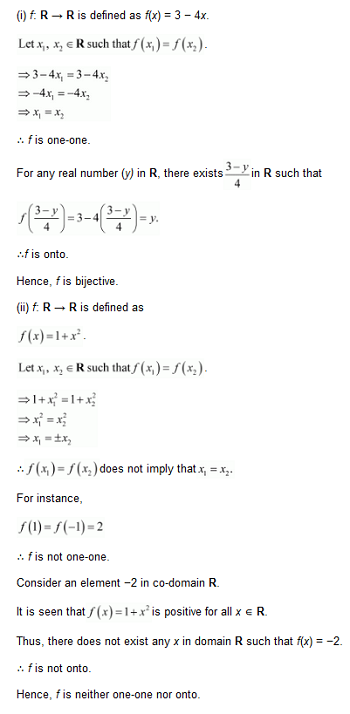Question8. Let A and B be sets. Show that f: A × B → B × A such that (a, b) = (b, a) is bijective function.

Solution :

Question9. Let N → N be  defined by f(n)  for all

Solution :

Question10. Let A = R – {3} and B = R – {1}. Consider the function f : A → B defined by f(x)  = (x-2/x-3) Is f one-one and onto? Justify your answer.

Solution :
A = R – {3} and B = R – {1}

f : A → B defined by f(x)  = (x-2/x-3)

Let x, y ∈ A such that f(x) =  f(y)

Hence, function f  is one-one and onto.

Question11. Let f: R → R be defined as f(x) = x4. Choose the correct answer.

(A) f is one-one onto

(B) f is many-one onto

(C) f is one-one but not onto

(D) f is neither one-one nor onto

Solution :

f: R→ R is defined as f(x) = x4.

Let ,x, y ∈ R  such that f(x) = f(y).

⇒ x4 = y4

⇒ x = ±y

∴f(x1) = f (x2)  does not imply that x1 = x2

For instance,

f(1) = f(-1) = 1

∴ f  is not one-one.

Consider an element 2 in co-domain R . It is clear that there does not exist any x  in domain  R  such that f(x) = 2.

∴ f  is not onto.

Hence, function f  is neither one-one nor onto.

Question12. Let f: R → R be defined as f(x) = 3x. Choose the correct answer.

(A) f is one-one onto

(B) f is many-one onto

(C) f is one-one but not onto

(D) f is neither one-one nor onto

Solution : f: R → R be defined as f(x) = 3x.

Let ,x, y ∈ R  such that f(x) = f(y).

⇒ 3x = 3y

⇒ x = y

∴ f is one – one

Also, for any real number (y)  in co-domain R, there exists y/3 in R  such that f(y/3) = 3(y/3) = y

∴ f is onto.

Hence, function f  is one-one and onto.

### Solve The Following Questions.

Question1. Let f: {1, 3, 4} → {1, 2, 5} and g: {1, 2, 5} → {1, 3} be given by f = {(1, 2), (3, 5), (4, 1)} and g = {(1, 3), (2, 3), (5, 1)}. Write down gof.

Solution :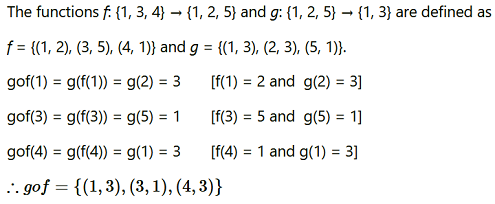Question2. Let f,g and h be functions from R to R. Show that:

(f + g)oh = foh + goh

(f.g)oh = (foh). (goh)

Solution :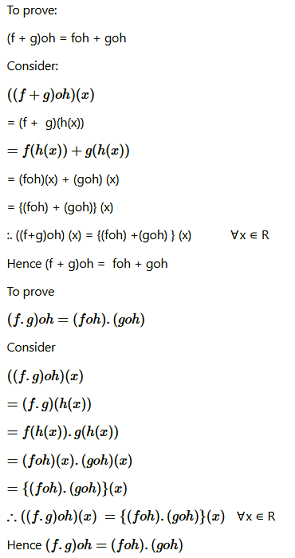Question3. Find gof and fog , if:

(i) f(x) = |x| and g(x) = |5x – 2|

(ii) f(x) = 8x3 and g(x) = x1/3

Solution :

Question4. If show that fof (x) = x for all x ≠ 2/3 What is the inverse of f

Solution :

Hence, the given function f  is invertible and the inverse of f is  itself.

Question5. State with reason whether following functions have inverse

(i) f: {1, 2, 3, 4} → {10} with f = {(1, 10), (2, 10), (3, 10), (4, 10)}

(ii) g: {5, 6, 7, 8} → {1, 2, 3, 4} with g = {(5, 4), (6, 3), (7, 4), (8, 2)}

(iii) h: {2, 3, 4, 5} → {7, 9, 11, 13} with h = {(2, 7), (3, 9), (4, 11), (5, 13)}

Solution :
(i) f: {1, 2, 3, 4} → {10}defined as:

f = {(1, 10), (2, 10), (3, 10), (4, 10)}

From the given definition of f, we can see that f is a many one function as: f(1) = f(2) = f(3) = f(4) = 10

∴f is not one-one.

Hence, function f does not have an inverse.

(ii) g: {5, 6, 7, 8} → {1, 2, 3, 4} defined as:

g = {(5, 4), (6, 3), (7, 4), (8, 2)}

From the given definition of g, it is seen that g is a many one function as: g(5) = g(7) = 4.

∴g is not one-one,

Hence, function g does not have an inverse.

(iii) h: {2, 3, 4, 5} → {7, 9, 11, 13} defined as:

h = {(2, 7), (3, 9), (4, 11), (5, 13)}

It is seen that all distinct elements of the set {2, 3, 4, 5} have distinct images under h.

∴Function h is one-one.

Also, h is onto since for every element y of the set {7, 9, 11, 13}, there exists an element x in the set {2, 3, 4, 5}such that h(x) = y.

Thus, h is a one-one and onto function. Hence, h has an inverse.

Question6. Show that f: [-1,1] → R given by f(x) =  x/x+2 is one-one. Find the inverse of the function f: [-1,1] → Range f

Solution :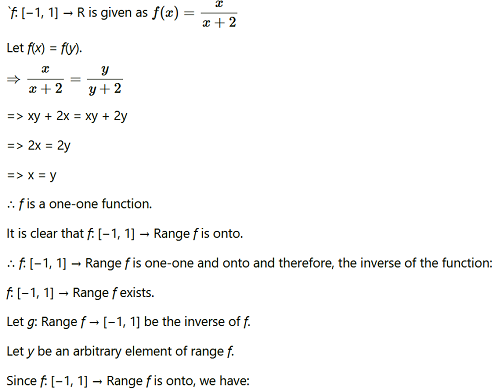Question7. Consider f : R→ R given by f(x) = 4x + 3 Show that f is invertible. Find the inverse of f.

Solution :

Question8. Consider f: R→ [4, ∞)  given by f(x) = x2 + 4 Show that f is invertible with the inverse f−1 of f given by f−1(y)  = √y – 4  where Ris the set of all non-negative real numbers.

Solution :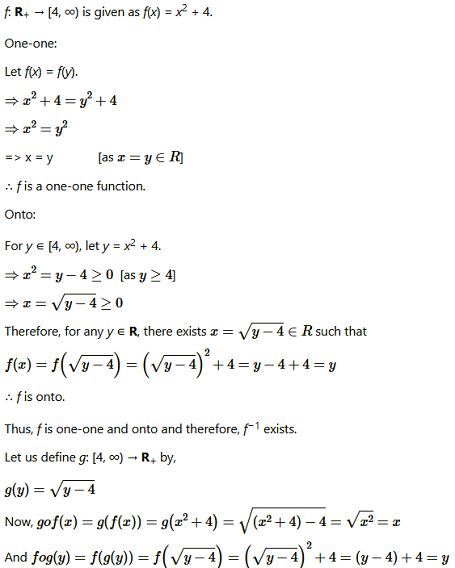Question9. Consider f: R+ → [−5, ∞) given by f(x) = 9x2 + 6x − 5. Show that f is invertible with

Solution :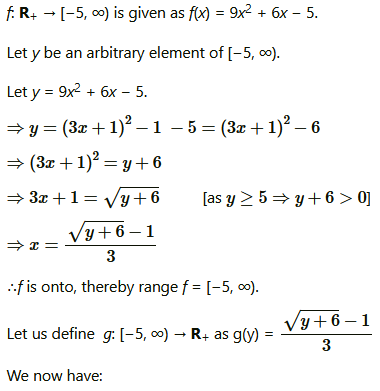Question10.  Let f: X → Y be an invertible function. Show that f has unique inverse.

(Hint: suppose g1 and g2 are two inverses of f. Then for all y ∈ Y, fog1(y) = IY(y) = fog2(y). Use one-one ness of f).

Solution :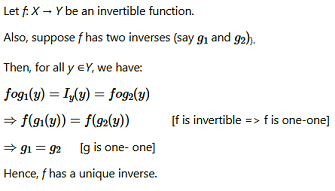Question11. Consider f: {1, 2, 3} → {a, b, c} given by f(1) = a, f(2) = b and f(3) = c. Find f−1 and show that (f−1)−1 = f.

Solution :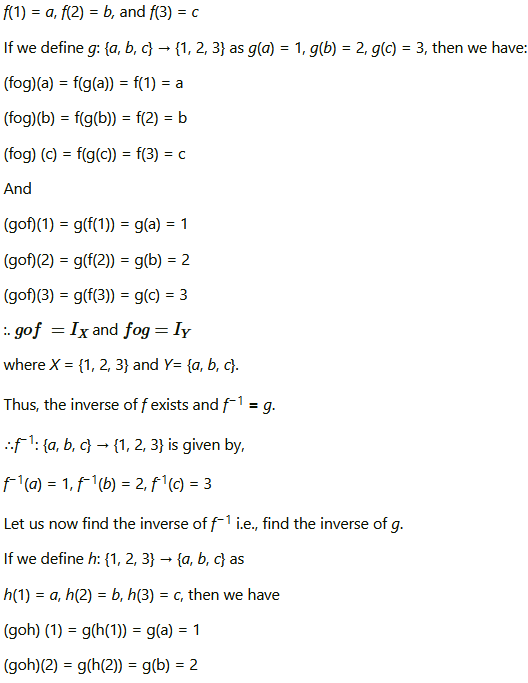Question12. Let f: X → Y be an invertible function. Show that the inverse of f−1 is f, i.e., (f−1)−1 = f.

Solution :
Let f: X → Y be an invertible function.

Then, there exists a function g: Y → X such that gof = IXand fog = IY.

Here, f−1 = g.

Now, gof = IX and fog = IY

⇒ f−1of = IX and fof−1= IY

Hence, f−1: Y → X is invertible and f is the inverse of f−1

i.e., (f−1)−1 = f.

Question13.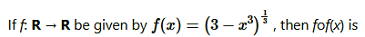(A) 1/x3

(B) x3

(C) x

(D) (3 − x3)

Solution :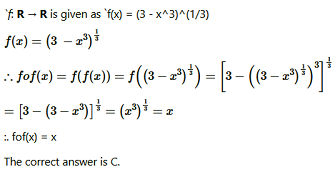Question14.

Solution :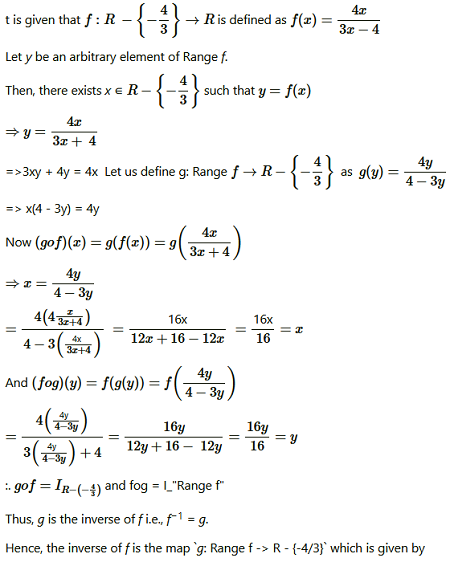### Solve The Following Questions.

Question1. Determine whether or not each of the definition of given below gives a binary operation. In the event that * is not a binary operation, give justification for this.

(i) On Z+, define * by a * b = a − b

(ii) On Z+, define * by a * b = ab

(iii) On R, define * by a * b = ab2

(iv) On Z+, define * by a * b = |a − b|

(v) On Z+, define * by a * b = a

Solution :
(i) On Z+, * is defined by a * b = a − b.

It is not a binary operation as the image of (1, 2) under * is 1 * 2 = 1 − 2
= −1 ∉ Z+.

(ii) On Z+, * is defined by a * b = ab.

It is seen that for each a, b ∈ Z+, there is a unique element ab in Z+

This means that * carries each pair (a, b) to a unique element a * b = ab in Z+.

Therefore, * is a binary operation.

(iii) On R, * is defined by a * b = ab2.

It is seen that for each a, b ∈ R, there is a unique element ab2 in R.

This means that * carries each pair (a, b) to a unique element a * b = ab2 in R.

Therefore, * is a binary operation.

(iv) On Z+, * is defined by a * b = |a − b|.

It is seen that for each a, b ∈ Z+, there is a unique element |a − b| in Z+

This means that * carries each pair (a, b) to a unique element a * b = |a − b| in Z+.

Therefore, * is a binary operation.

(v) On Z+, * is defined by a * b = a.

* carries each pair (a, b) to a unique element a * b = a in Z+.

Therefore, * is a binary operation.

Question2. For each binary operation * defined below, determine whether * is commutative or associative:

(i) On Z, define − b

(ii) On Q, define ab + 1

(iii) On Q, define =ab/2

(iv) On Z+, define = 2ab

(v) On Z+, define ab

(vi) On R − {−1}, define  a.b = a/b+1

Solution :

(i) On Z, * is defined by a * b = a − b.

It can be observed that 1 * 2 = 1 − 2 = 1 and 2 * 1 = 2 − 1 = 1.

∴1 * 2 ≠ 2 * 1; where 1, 2 ∈ Z

Hence, the operation * is not commutative.

Also we have:

(1 * 2) * 3 = (1 − 2) * 3 = −1 * 3 = −1 − 3 = −4

1 * (2 * 3) = 1 * (2 − 3) = 1 * −1 = 1 − (−1) = 2

∴(1 * 2) * 3 ≠ 1 * (2 * 3) ; where 1, 2, 3 ∈ Z

Hence, the operation * is not associative.

(ii) On Q, * is defined by * b = ab + 1.

It is known that:

ab = ba &mnForE; a, b ∈ Q

⇒ ab + 1 = ba + 1 &mnForE; a, b ∈ Q

⇒ * b = * b &mnForE; a, b ∈ Q

Therefore, the operation * is commutative.

It can be observed that:

(1 * 2) * 3 = (1 × 2 + 1) * 3 = 3 * 3 = 3 × 3 + 1 = 10

1 * (2 * 3) = 1 * (2 × 3 + 1) = 1 * 7 = 1 × 7 + 1 = 8

∴(1 * 2) * 3 ≠ 1 * (2 * 3) ; where 1, 2, 3 ∈ Q

Therefore, the operation * is not associative.

(iii) On Q, * is defined by * b = ab/2

It is known that:

ab = ba &mnForE; a, b ∈ Q

⇒ ab/2 = ba/2 &mn ForE; a, b ∈ Q

⇒ * b = * a &mnForE; a, b ∈ Q

Therefore, the operation * is commutative.

For all a, b, c ∈ Q, we have:

Therefore, the operation * is associative.

(iv) On Z+, * is defined by * b = 2ab.

It is known that:

ab = ba &mnForE; a, b ∈ Z+

⇒ 2ab = 2ba &mnForE; a, b ∈ Z+

⇒ * b = * a &mnForE; a, b ∈ Z+

Therefore, the operation * is commutative.

It can be observed that:

∴(1 * 2) * 3 ≠ 1 * (2 * 3) ; where 1, 2, 3 ∈ Z+

Therefore, the operation * is not associative.

(v) On Z+, * is defined by * b = ab.

It can be observed that:

1 x2 = 12=1 and 2x 1 =21 = 2

∴ 1 * 2 ≠ 2 * 1 ; where 1, 2 ∈ Z+

Therefore, the operation * is not commutative.

It can also be observed that:

∴(2 * 3) * 4 ≠ 2 * (3 * 4) ; where 2, 3, 4 ∈ Z+

Therefore, the operation * is not associative.

(vi) On R, * − {−1} is defined by a.b = a/b+1

It can be observed that 1 x 2 = 1/2+1 = 1/2 and 2 x 1 = 2/1+1 = 2/2

∴1 * 2 ≠ 2 * 1 ; where 1, 2 ∈ − {−1}

Therefore, the operation * is not commutative.

It can also be observed that:

∴ (1 * 2) * 3 ≠ 1 * (2 * 3) ; where 1, 2, 3 ∈ − {−1}

Therefore, the operation * is not associative.

Question3. Consider the binary operation ∨ on the set {1, 2, 3, 4, 5} defined by a ∨b = min {a, b}. Write the operation table of the operation∨.

Solution :
The binary operation ∨ on the set {1, 2, 3, 4, 5} is defined as a ∨ b = min {a, b} & mn For E; a, b ∈ {1, 2, 3, 4, 5}.

Thus, the operation table for the given operation ∨ can be given as:

4. Consider a binary operation * on the set {1, 2, 3, 4, 5} given by the following multiplication table.

(i) Compute (2 * 3) * 4 and 2 * (3 * 4)

(ii) Is * commutative?

(iii) Compute (2 * 3) * (4 * 5).

(Hint: use the following table)

Solution :
(i) (2 * 3) * 4 = 1 * 4 = 1

2 * (3 * 4) = 2 * 1 = 1

(ii) For every a, b ∈{1, 2, 3, 4, 5}, we have a * b = b * a. Therefore, the operation * is commutative.

(iii) (2 * 3) = 1 and (4 * 5) = 1

∴(2 * 3) * (4 * 5) = 1 * 1 = 1

Question5. Let*′ be the binary operation on the set {1, 2, 3, 4, 5} defined by a *′ b = H.C.F. of a and b. Is the operation *′ same as the operation * defined in Exercise 4 above? Justify your answer.

Solution :
The binary operation *′ on the set {1, 2, 3 4, 5} is defined as a *′ b = H.C.F of a and b.

The operation table for the operation *′ can be given as:

We observe that the operation tables for the operations * and *′ are the same.

Thus, the operation *′ is same as the operation*.

Question6. Let * be the binary operation on N given by a * b = L.C.M. of a and b. Find

(i) 5 * 7, 20 * 16 (ii) Is * commutative?

(iii) Is * associative? (iv) Find the identity of * in N

(v) Which elements of N are invertible for the operation *?

Solution :
The binary operation * on N is defined as a * b = L.C.M. of a and b.

(i) 5 * 7 = L.C.M. of 5 and 7 = 35

20 * 16 = L.C.M of 20 and 16 = 80

(ii) It is known that:

L.C.M of a and b = L.C.M of b and a &mnForE; a, b ∈ N.

∴a * b = b * a

Thus, the operation * is commutative.

(iii) For a, b, c ∈ N, we have:

(a * b) * c = (L.C.M of a and b) * c = LCM of a, b, and c

a * (b * c) = a * (LCM of b and c) = L.C.M of a, b, and c

∴(a * b) * c = a * (b * c)

Thus, the operation * is associative.

(iv) It is known that:

L.C.M. of a and 1 = a = L.C.M. 1 and a &mnForE; a ∈ N

⇒ a * 1 = a = 1 * a &mnForE; a ∈ N

Thus, 1 is the identity of * in N.

(v) An element a in N is invertible with respect to the operation * if there exists an element b in N, such that a * b = e = b * a.

Here, e = 1

This means that:

L.C.M of a and b = 1 = L.C.M of b and a

This case is possible only when a and b are equal to 1.

Thus, 1 is the only invertible element of N with respect to the operation *.

Question7. Is * defined on the set {1, 2, 3, 4, 5} by a * b = L.C.M. of a and b a binary operation? Justify your answer.

Solution :
The operation * on the set A = {1, 2, 3, 4, 5} is defined as

a * b = L.C.M. of a and b.

Then, the operation table for the given operation * can be given as:

It can be observed from the obtained table that:

3 * 2 = 2 * 3 = 6 ∉ A, 5 * 2 = 2 * 5 = 10 ∉ A, 3 * 4 = 4 * 3 = 12 ∉ A

3 * 5 = 5 * 3 = 15 ∉ A, 4 * 5 = 5 * 4 = 20 ∉ A

Hence, the given operation * is not a binary operation.

Question8. Let * be the binary operation on N defined by a * b = H.C.F. of a and b. Is * commutative? Is * associative? Does there exist identity for this binary operation on N?

Solution :
The binary operation * on N is defined as:

a * b = H.C.F. of a and b

It is known that:

H.C.F. of a and b = H.C.F. of b and a &mnForE; a, b ∈ N.

∴a * b = b * a

Thus, the operation * is commutative.

For a, b, c ∈ N, we have:

(a * b)* c = (H.C.F. of a and b) * c = H.C.F. of a, b, and c

a *(b * c)= a *(H.C.F. of b and c) = H.C.F. of a, b, and c

∴(a * b) * c = a * (b * c)

Thus, the operation * is associative.

Now, an element e ∈ N will be the identity for the operation * if a * e = a = e* a ∈ a ∈ N.

But this relation is not true for any a ∈ N.

Thus, the operation * does not have any identity in N.

Question9. Let * be a binary operation on the set Q of rational numbers as follows:

(i) a * b = a − b

(ii) a * b = a2 + b2

(iii) a * b = a + ab

(iv) a * b = (a − b)2

(v) a * b = ab/4  (vi) a * b = ab2

Find which of the binary operations are commutative and which are associative.

Ans.

10. Find which of the operations given above has identity.

Solution :
Let the identity be I.

Question 11. Let A = N × N and * be the binary operation on A defined by

(a, b) * (c, d) = (a + c, b + d)

Show that * is commutative and associative. Find the identity element for * on A, if any.

Solution :
A = N × N

* is a binary operation on A and is defined by:

(a, b) * (c, d) = (a + c, b + d)

Let (a, b), (c, d) ∈ A

Then, a, b, c, d ∈ N

We have:

(a, b) * (c, d) = (a + c, b + d)

(c, d) * (a, b) = (c + a, d + b) = (a + c, b + d)

[Addition is commutative in the set of natural numbers]

∴(a, b) * (c, d) = (c, d) * (a, b)

Therefore, the operation * is commutative.

Now, let (a, b), (c, d), (e, f) ∈A

Then, a, b, c, d, e, f ∈ N

We have:

Question12. State whether the following statements are true or false. Justify.

(i) For an arbitrary binary operation * on a set N, a ∀ * N.

(ii) If * is a commutative binary operation on N, then a * (b * c) = (c * b) * a

Solution :
(i) Define an operation * on N as:

a * b = a + b  a, b ∈ N

Then, in particular, for b = a = 3, we have:

3 * 3 = 3 + 3 = 6 ≠ 3

Therefore, statement (i) is false.

(ii) R.H.S. = (c * b) * a

= (b * c) * a [* is commutative]

= a * (b * c) [Again, as * is commutative]

= L.H.S.

∴ a * (b * c) = (c * b) * a

Therefore, statement (ii) is true.

Question13. Consider a binary operation * on N defined as a * b = a3 + b3. Choose the correct answer.

(A) Is * both associative and commutative?

(B) Is * commutative but not associative?

(C) Is * associative but not commutative?

(D) Is * neither commutative nor associative?

Solution :
On N, the operation * is defined as a * b = a3 + b3.

For, a, b, ∈ N, we have:

a * b = a3 + b3 = b3 + a= b * a [Addition is commutative in N]

Therefore, the operation * is commutative.

It can be observed that:

(1*2)*3 = (13+23)*3 = 9 * 3 = 93 + 33 = 729 + 27 = 756

Also, 1*(2*3) = 1*(2+33) = 1*(8 +27) = 1 × 35

= 13 +353 = 1 +(35)3 = 1 + 42875 = 42876.

∴(1 * 2) * 3 ≠ 1 * (2 * 3) ; where 1, 2, 3 ∈ N

Therefore, the operation * is not associative.

Hence, the operation * is commutative, but not associative. Thus, the correct answer is B.

### Solve The Following Questions.

Question1. Let f: R → R be defined as f(x) = 10x + 7. Find the function g: R → R such that g o f = f o g = 1R.

Solution :

Question2. Let f: W → W be defined as f(n) = n − 1, if is odd and f(n) = n + 1, if n is even. Show that f is invertible. Find the inverse of f. Here, W is the set of all whole numbers.

Solution :

Question3. If f: R → R is defined by f(x) = x2 − 3x + 2, find f(f(x)).

Solution :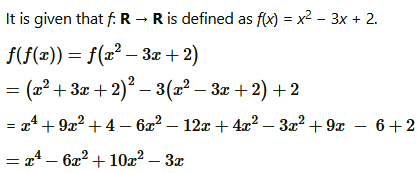Question4. Show that function f: R → {x ∈ R: −1 < x < 1} defined by f(x) = x/1+|x| x ∈R is one-one and onto function.

Solution :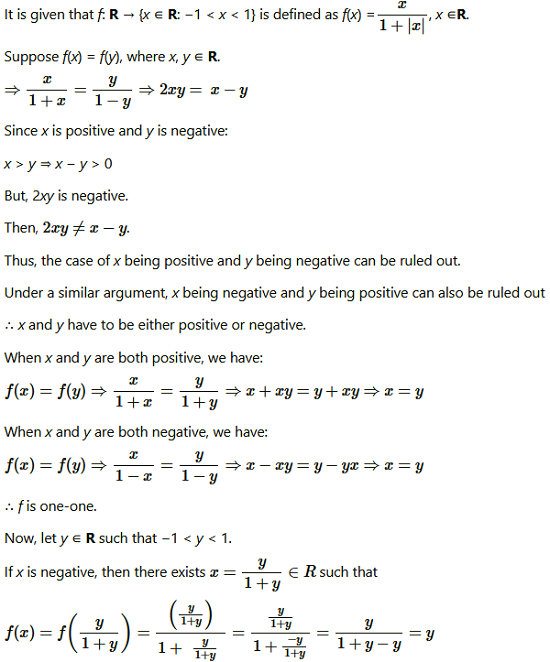Question5. Show that the function f: R → R given by f(x) = x3 is injective.

Ans f: R → R is given as f(x) = x3.

Suppose f(x) = f(y), where x, y ∈ R.

⇒ x3 = y3 … (1)

Now, we need to show that x = y.

Suppose x ≠ y, their cubes will also not be equal.

⇒ x3 ≠ y3

However, this will be a contradiction to (1).

∴ x = y

Hence, f is injective.

Question6. Give examples of two functions f: N → Z and g: Z → Z such that g o f is injective but g is not injective.

(Hint: Consider f(x) = x and g(x) = |x| )

Solution :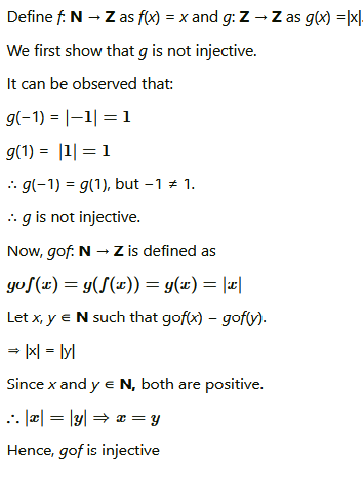Question 7. Given examples of two functions f: N → N and g: N → N such that gof is onto but f is not onto.

(Hint: Consider f(x) = x + 1 and )

Solution :

Question8. Given a non empty set X, consider P(X) which is the set of all subsets of X.

Define the relation R in P(X) as follows: For subsets A, B in P(X), ARB if and only if A ⊂ B. Is R an equivalence relation on P(X)? Justify you answer:

Solution :
Since every set is a subset of itself, ARA for all A ∈ P(X).

∴R is reflexive.

Let ARB ⇒ A ⊂ B.

This cannot be implied to B ⊂ A.

For instance, if A = {1, 2} and B = {1, 2, 3}, then it cannot be implied that B is related to A.

∴ R is not symmetric.

Further, if ARB and BRC, then A ⊂ B and B ⊂ C.

⇒ A ⊂ C

⇒ ARC

∴ R is transitive.

Hence, R is not an equivalence relation since it is not symmetric.

Question9. Given a non-empty set X, consider the binary operation *: P(X) × P(X) → P(X) given by A * B = A ∩ B & mn For  E; A, B in P(X) is the power set of X. Show that X is the identity element for this operation and X is the only invertible element in P(X) with respect to the operation*.

Solution :
Let S be a non-empty set and P(S) be its power set. Let any two subsets A and B of S.

Question10. Find the number of all onto functions from the set {1, 2, 3, … , n) to itself.

Solution :
Onto functions from the set {1, 2, 3, … ,n} to itself is simply a permutation on n symbols 1, 2, …, n.

Thus, the total number of onto maps from {1, 2, … , n} to itself is the same as the total number of permutations on n symbols 1, 2, …, n, which is n!.

11. Let S = {a, b, c} and T = {1, 2, 3}. Find F−1 of the following functions F from S to T, if it exists.

(i) F = {(a, 3), (b, 2), (c, 1)} (ii) F = {(a, 2), (b, 1), (c, 1)}

Solution :
S = {a, b, c}, T = {1, 2, 3}

(i) F: S → T is defined as:

F = {(a, 3), (b, 2), (c, 1)}

⇒ F (a) = 3, F (b) = 2, F(c) = 1

Therefore, F−1: T → S is given by

F−1 = {(3, a), (2, b), (1, c)}.

(ii) F: S → T is defined as:

F = {(a, 2), (b, 1), (c, 1)}

Since F (b) = F (c) = 1, F is not one-one.

Hence, F is not invertible i.e., F−1 does not exist.

Question12. Consider the binary operations*: R ×R → and o: R × R → R defined as a * b  = |a – b| and  ao b = a, & mn For E; a, b ∈ R. Show that * is commutative but not associative, o is associative but not commutative. Further, show that &mn For E;a, b, c ∈ R, a*(b o c) = (a * b) o (a * c). [If it is so, we say that the operation * distributes over the operation o]. Does o distribute over *? Justify your answer.

Solution :

Question13. Given a non-empty set X, let *: P(X) × P(X) → P(X) be defined as A * B = (A − B) ∪ (B − A), & mn For E; A, B ∈ P(X). Show that the empty set Φ is the identity for the operation * and all the elements A of P(X) are invertible with A−1 = A. (Hint: (A − Φ) ∪ (Φ − A) = A and (A − A) ∪ (A − A) = A * A = Φ).

Solution :
: It is given that ∗: P(X) × P(X) → P(X) be defined as

A * B = (A – B) ∪ (B – A), A, B ∈ P(X).

Now, let A ϵ P(X). Then, we get,

A * ф = (A – ф) ∪ (ф –A) = A∪ф = A

ф * A = (ф – A) ∪ (A – ф ) = ф∪A = A

A * ф = A = ф * A,    A ϵ P(X)

Therefore, ф is the identity element for the given operation *.

Now, an element A ϵ P(X) will be invertible if there exists B ϵ P(X) such that

A * B = ф = B * A. (as ф is an identity element.)

Now, we can see that A * A = (A –A) ∪ (A – A) = ф∪ф = ф AϵP(X).

Therefore, all the element A of P(X) are invertible with A-1 = A.

Question14. Define binary operation * on the set {0, 1, 2, 3, 4, 5} as

Show that zero is the identity for this operation and each element a ≠ 0 of the set is invertible with 6 – abeing the inverse of a

Solution :

Question15. Let A = {–1, 0, 1, 2}, B = {–4, –2, 0, 2} and f; g: A→B be the functions defined by f(x) = x2 − xx ∈  A and g(x) = 2x – 1/2 – 1. x ∈  A. Are f and g equal? Justify your answer.

(Hint: One may note that two functions fA → B and g: A → B such that f(a) = g(a) &mnForE;a ∈A,, are called equal functions).

Solution :

Question16. Let A = {1, 2, 3}. Then number of relations containing (1, 2) and (1, 3) which are reflexive and symmetric but not transitive is:

(A) 1

(B) 2

(C) 3

(D) 4

Solution :
It is clear that 1 is reflexive and symmetric but not transitive.

Therefore, option (A) is correct.

Question17. Let A = {1, 2, 3}. Then number of equivalence relations containing (1, 2) is:

(A) 1

(B) 2

(C) 3

(D) 4

Solution :
2, Therefore, option (B) is correct.

Question18. Let R → R be the Signum Function defined as and R → R be the Greatest Function given by g(x) = [x] where [x] is greatest integer less than or equal to x

Then, does fog and gof  coincide in (0, 1)?

Solution :

Therefore, option (B) is correct.

Question19. Number of binary operation on the set {a, b} are:

(A) 10

(b) 16

(C) 20

(D) 8

Solution :
A binary operation * on {a, b} is a function from {a, b} × {a, b} → {a, b}

i.e., * is a function from {(a, a), (a, b), (b, a), (b, b)} → {a, b}.

Hence, the total number of binary operations on the set {a, b} is 24 i.e., 16.